Related Articles

# MATLAB – Data Types

• Last Updated : 04 Jul, 2021

MATLAB is a platform which provides millions of Engineers and Scientists to analyze data using programming and numerical computing algorithm and also help in creating models. Data types are particular types of data items defined by the values they can store in them, generally, in programming languages they are used.

Define data types in MATLAB

In MATLAB we do not require any type of declaration statement, when it gets any new variable name it creates the variable and allocates appropriate memory space to it but if the variable name already exists it will replace the original content with new content and allocate it to new storage space when required.

Syntax: variable name = a value (or an expression)

Example:

## Matlab

 `% MATLAB code for variable initialization ``Geeks = 7;`

Output: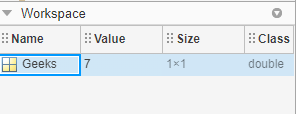### Data Types in MATLAB

In MATLAB data can be stored in different types, numeric, text, complex number, etc. To store these data MATLAB has different classes which have various characteristics. MATLAB provides a total of 16 fundamental data types.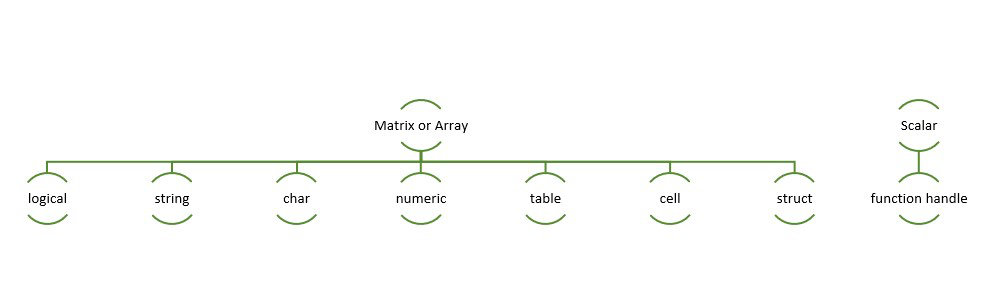### Logic Type

Logic types are True and false values that are represented with the logical value 0 and 1. Any numerical value (non-complex) can be converted into a logical representation.

Syntax:G = logical (x)

Example:

## Matlab

 `% MATLAB code for random matrix generation``rng default``A = randi(5,5) ``% It will generate random matrix of 15x5`` ` `B = A < 9 ``% The result is a logical matrix.``% Each value in B represents a logical 1 (true) ``% or logical 0 (false) state to indicate whether``% the corresponding element of A fulfills the condition A < 9. ``% For example, A(1,1) is 13, so B(1,1) is logical 0 (false). ``% However, A(1,2) is 2, so B(1,2) is logical 1 (true).`

Output: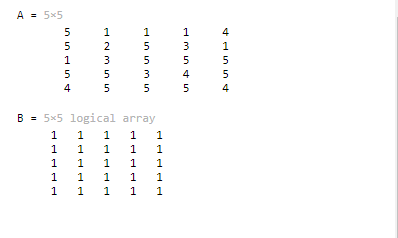### Char and String type

In MATLAB character and string array provide storage for text type data. The strings are character array compared with the sequence of numbers called a numeric array.

Syntax: s = ‘String’

Example:

## Matlab

 `% MATLAB code for showing String results``str = ``"Welcome to GeeksforGeeks, "``"Welcome!"``" and lets start coding."``fprintf(str);`

Output: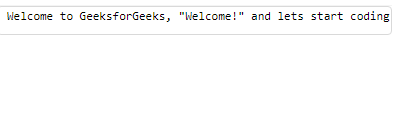### Numeric Type-

Integer and floating-point data are in this type with the following descriptions.

Example:

## Matlab

 `% MATLAB code for numeric type``str = ``'Hello World'``;``int8(str)`

Output: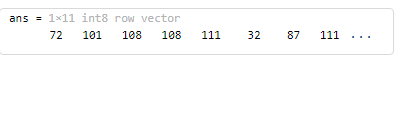### Table

The table contains rows and column variables. Each variable can be of different data types and different sizes, but each variable needs to have the same number of rows. Range of functions are used to access data to create, edit, and read the table data.

Syntax:T = table(ColumnName1,ColumnName2);

Example:

## Matlab

 `% MATLAB code for Table ``T = table(Name,QuestionAttempted,CodingScore);``data = {``'Atul Sisodiya'``,22,100};``Tnew = [Tnew;data];`

Output:

```Table array
2x3
Name             QuestionAttempted          CodingScore
Atul Sisodiya                   22                100```

### Cell

A cell array is a MATLAB data type that contains indexed data containers called cells. Cells can contain any type of data, commonly contain character vectors of different lengths, numbers, an array of numbers of any size. Sets of cells are enclosed in () and access to the cells is done by using {} which is to create, edit or delete any cell functions.

Syntax: c = { }

Example:

## Matlab

 `C = {1, 2, 3}`

Output: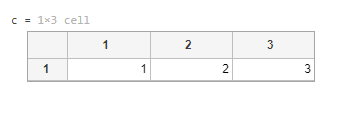### Structure

In structure data containers are used to group related data and their type, which are called fields. Fields may contain any type of data. In structures, Data is accessed using the dot notation.

Syntax: structname.fieldName

Example:

## Matlab

 `geek(1).name = (``"Atul Sisodiya"``);``geek(1).coding = 100;``geek`

Output: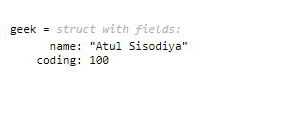### Function Handles

Function Handles is majorly used in MATLAB is to pass a function (numerical or char) to another function. Variables that are used to invoke function indirectly can be named as a Function handle.

To create a function handle ‘@’ operator is used.

Example: To create a function handle to evaluate x^2 + y^2, a function used is:

Output: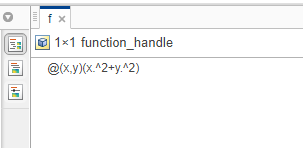My Personal Notes arrow_drop_up